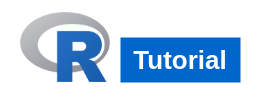## R Tutorial

We present you the R Tutorial, to learn R, the basics of R programming language, interfacing data to R from different data sources, creating charts and graphs, and extracting statistical information.### What is R programming language ?

R is an open source programming language. It has become one of the powerful choices for statistical analysis. R helps you to get big picture of your data by calculating statistical parameters like mean, standard deviation, correlation etc.

### Features of R

• Open Source and Free to use.
• Works well for statistics.
• Command-Line Programming Language – But IDEs like R Studio and plugins to the popular IDEs like Eclipse, etc., are available.
• Easy sharing of Results or Analysis.
• Integration with other packages and programming languages.
• R programming is easy and informative.

### R Tutorial Index

In our R Tutorial, we shall take you through the following topics :

### Data Interfacing – from different sources of data to R language

• R CSV Files – Learn R functions to read CSV Files, analyze or filter data read from CSV Files, and write back filtered data to CSV Files.
• R Read Excel XLS XLSX Files
• R Binary Files
• R XML Files
• R JSON Files
• R Web Data
• R Database

### Statistical Analysis

• R Mean of a Vector
• R Median of a Vector
• R Mode
• R Linear Regression
• R Multiple Regression
• R Logistic Regression
• R Normal Distribution
• R Binomial Distribution
• R Poisson Regression
• R Analysis of Covariance
• R Time Series Analysis
• R Nonlinear Least Square
• R Decision Tree
• R Random Forest
• R Survival Analysis
• R Chi Square Tests

### Conclusion :

With this R Tutorial, we have learnt the basics of R, how to interface data to R from different sources, create charts and graphs, and extract statistical information.